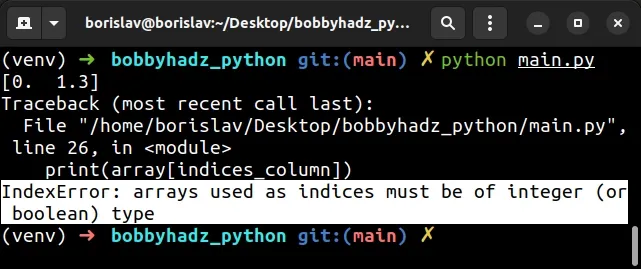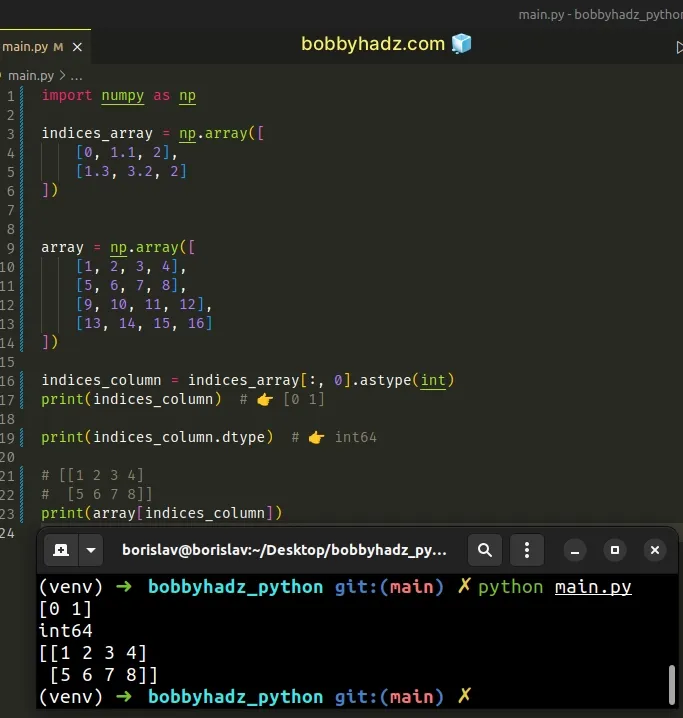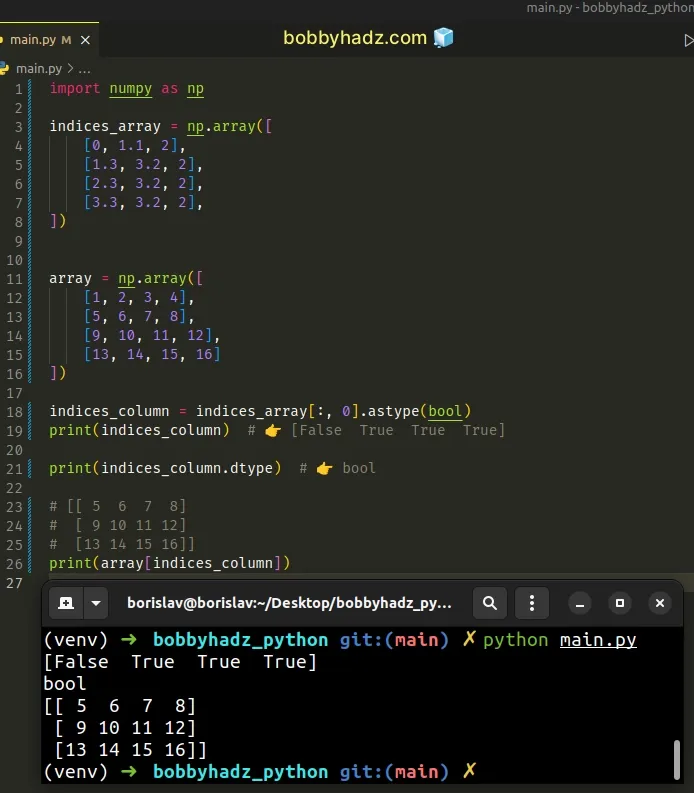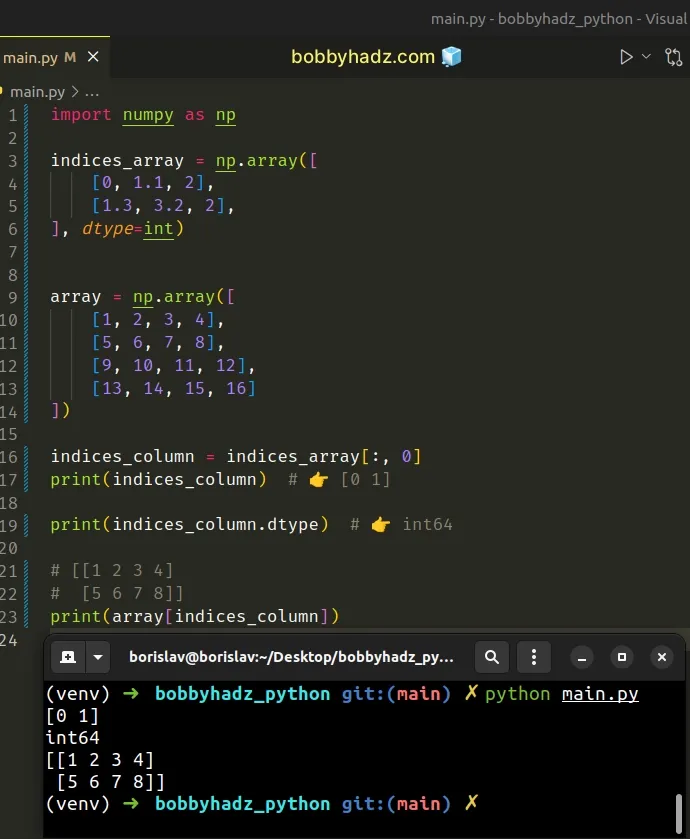# Arrays used as indices must be of integer (or boolean) typeLast updated: Jul 6, 2023
4 min## #Arrays used as indices must be of integer (or boolean) type

The NumPy "IndexError: arrays used as indices must be of integer (or boolean) type" occurs when you try to use an array containing non-integer or boolean values when indexing another array.

To solve the error, use the `astype()` method to convert the type of the array elements to integers before using it to index another array.

Here is an example of how the error occurs.

main.py
```Copied!```import numpy as np

indices_array = np.array([
[0, 1.1, 2],
[1.3, 3.2, 2]
])

array = np.array([
[1, 2, 3, 4],
[5, 6, 7, 8],
[9, 10, 11, 12],
[13, 14, 15, 16]
])

indices_column = indices_array[:, 0]
print(indices_column)  # 👉️ [0.  1.3]

# ⛔️ IndexError: arrays used as indices must be of integer (or boolean) type
print(array[indices_column])
``````The `indices_array` variable stores an array that we will use the index the other array.

However, notice that some of the values in the indices array are of type float.

main.py
```Copied!```import numpy as np

indices_array = np.array([
[0, 1.1, 2],
[1.3, 3.2, 2]
])

# [[0.  1.1 2. ]
#  [1.3 3.2 2. ]]
print(indices_array)
``````

You can use the dtype attribute to check the type of the values in the NumPy array.

main.py
```Copied!```import numpy as np

indices_array = np.array([
[0, 1.1, 2],
[1.3, 3.2, 2]
])

print(indices_array.dtype) # 👉️ float64
``````

Notice that the type of the values in the array is `float64`.

The values in the array have to either be integers or booleans for us to be able to use the array to index another array.

## #Using the `astype()` method to solve the error

One way to solve the error is to use the `astype()` method to convert the values in the array to integers.

main.py
```Copied!```import numpy as np

indices_array = np.array([
[0, 1.1, 2],
[1.3, 3.2, 2]
])

array = np.array([
[1, 2, 3, 4],
[5, 6, 7, 8],
[9, 10, 11, 12],
[13, 14, 15, 16]
])

indices_column = indices_array[:, 0].astype(int)
print(indices_column)  # 👉️ [0 1]

print(indices_column.dtype)  # 👉️ int64

# [[1 2 3 4]
#  [5 6 7 8]]
print(array[indices_column])
``````We used array slicing to get the first column of the indices array and called the ndarray.astype method on it.

The method copies the array and casts it to the specified type.

You can use the dtype attribute to verify that the indices array contains integer values.

main.py
```Copied!```indices_column = indices_array[:, 0].astype(int)
print(indices_column)  # 👉️ [0 1]

print(indices_column.dtype)  # 👉️ int64
``````

The values in the array have to either be of type `int` or `bool`.

If you convert the values in the indices array to booleans, `0` of any numeric type gets converted to `False`.

main.py
```Copied!```import numpy as np

indices_array = np.array([
[0, 1.1, 2],
[1.3, 3.2, 2],
[2.3, 3.2, 2],
[3.3, 3.2, 2],
])

array = np.array([
[1, 2, 3, 4],
[5, 6, 7, 8],
[9, 10, 11, 12],
[13, 14, 15, 16]
])

indices_column = indices_array[:, 0].astype(bool)
print(indices_column)  # 👉️ [False  True  True  True]

print(indices_column.dtype)  # 👉️ bool

# [[ 5  6  7  8]
#  [ 9 10 11 12]
#  [13 14 15 16]]
print(array[indices_column])
``````When you pass the `bool` type to the `astype` method, only `0` (zero) values of any numeric type get converted to `False`, assuming you have a numeric array.

If your array contains values of other types, then all falsy values will get converted to `False`.

The falsy values in Python are:

• constants defined to be falsy: `None` and `False`.
• `0` (zero) of any numeric type
• empty sequences and collections: `""` (empty string), `()` (empty tuple), `[]` (empty list), `{}` (empty dictionary), `set()` (empty set), `range(0)` (empty range).

All other values are truthy.

## #Setting the `dtype` of the indices array to `int` upon initialization

You can also set the `dtype` of the indices array to `int` upon initialization to solve the error.

main.py
```Copied!```import numpy as np

indices_array = np.array([
[0, 1.1, 2],
[1.3, 3.2, 2],
], dtype=int)

array = np.array([
[1, 2, 3, 4],
[5, 6, 7, 8],
[9, 10, 11, 12],
[13, 14, 15, 16]
])

indices_column = indices_array[:, 0]
print(indices_column)  # 👉️ [0 1]

print(indices_column.dtype)  # 👉️ int64

# [[1 2 3 4]
#  [5 6 7 8]]
print(array[indices_column])
``````Notice that we set the `dtype` argument to `int` when creating the indices array.

The numpy.array method takes an optional `dtype` argument.

The argument is used to specify the desired data type for the array.

If you don't explicitly supply the `dtype` argument, NumPy tries to use a default `dtype` that can represent the values in the array.